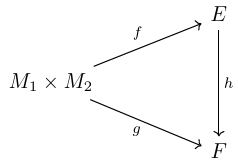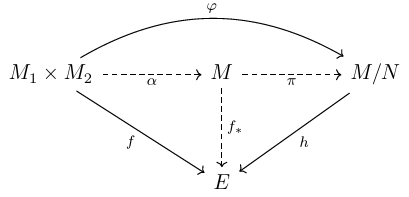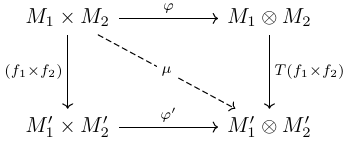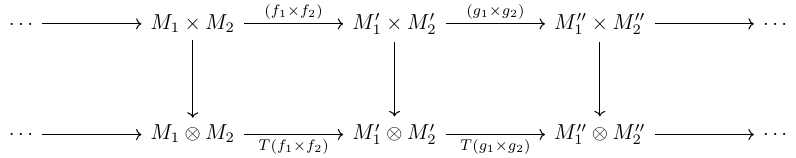Tensor Product as a Universal Object (Category Theory & Module Theory)

## Introduction

It is quite often to see direct sum or direct product of groups, modules, vector spaces. Indeed, for modules over a ring $R$, direct products are also direct products of $R$-modules as well. On the other hand, the direct sum is a coproduct in the category of $R$-modules.

But what about tensor products? It is some different kind of product but how? Is it related to direct product? How do we write a tensor product down? We need to solve this question but it is not a good idea to dig into numeric works.

## The category of bilinear or even $n$-multilinear maps

From now on, let $R$ be a commutative ring, and $M_1,\cdots,M_n$ are $R$-modules. Mainly we work on $M_1$ and $M_2$, i.e. $M_1 \times M_2$ and $M_1 \otimes M_2$. For $n$-multilinear one, simply replace $M_1\times M_2$ with $M_1 \times M_2 \times \cdots \times M_n$ and $M_1 \otimes M_2$ with $M_1 \otimes \cdots \otimes M_n$. The only difference is the change of symbols.

The bilinear maps of $M_1 \times M_2$ determines a category, say $BL(M_1 \times M_2)$ or we simply write $BL$. For an object $(f,E)$ in this category we have $f: M_1 \times M_2 \to E$ as a bilinear map and $E$ as a $R$-module of course. For two objects $(f,E)$ and $(g,F)$, we define the morphism between them as a linear function making the following diagram commutative: $\def\mor{\operatorname{Mor}}$This indeed makes $BL$ a category. If we define the morphisms from $(f,E)$ to $(g,F)$ by $\mor(f,g)$ (for simplicity we omit $E$ and $F$ since they are already determined by $f$ and $g$) we see the composition

satisfy all axioms for a category:

CAT 1 Two sets $\mor(f,g)$ and $\mor(f’,g’)$ are disjoint unless $f=f’$ and $g=g’$, in which case they are equal. If $g \neq g’$ but $f = f’$ for example, for any $h \in \mor(f,g)$, we have $g = h \circ f = h \circ f’ \neq g’$, hence $h \notin \mor(f,g)$. Other cases can be verified in the same fashion.

CAT 2 The existence of identity morphism. For any $(f,E) \in BL$, we simply take the identity map $i:E \to E$. For $h \in \mor(f,g)$, we see $g = h \circ f = h \circ i \circ f$. For $h’ \in \mor(g,f)$, we see $f = h’ \circ g = i \circ h’ \circ g$.

CAT 3 The law of composition is associative when defined.

There we have a category. But what about the tensor product? It is defined to be initial (or universally repelling) object in this category. Let’s denote this object by $(\varphi,M_1 \otimes M_2)$.

For any $(f,E) \in BL$, we have a unique morphism (which is a module homomorphism as well) $h:(\varphi,M_1 \otimes M_2) \to (f,E)$. For $x \in M_1$ and $y \in M_2$, we write $\varphi(x,y)=x \otimes y$. We call the existence of $h$ the universal property of $(\varphi,M_1 \otimes M_2)$.

The tensor product is unique up to isomorphism. That is, if both $(f,E)$ and $(g,F)$ are tensor products, then $E \simeq F$ in the sense of module isomorphism. Indeed, let $h \in \mor(f,g)$ and $h’ \in \mor(g,h)$ be the unique morphisms respectively, we see $g = h \circ f$, $f = h’ \circ g$, and therefore

Hence $h \circ h’$ is the identity of $(g,F)$ and $h’ \circ h$ is the identity of $(f,E)$. This gives $E \simeq F$.

What do we get so far? For any modules that is connected to $M_1 \times M_2$ with a bilinear map, the tensor product $M_1 \oplus M_2$ of $M_1$ and $M_2$, is always able to be connected to that module with a unique module homomorphism. What if there are more than one tensor products? Never mind. All tensor products are isomorphic.

But wait, does this definition make sense? Does this product even exist? How can we study the tensor product of two modules if we cannot even write it down? So far we are only working on arrows, and we don’t know what is happening inside an module. It is not a good idea to waste our time on ‘nonsenses’. We can look into it in an natural way. Indeed, if we can find a module satisfying the property we want, then we are done, since this can represent the tensor product under any circumstances. Again, all tensor products of $M_1$ and $M_2$ are isomorphic.

## A natural way to define the tensor product

Let $M$ be the free module generated by the set of all tuples $(x_1,x_2)$ where $x_1 \in M_1$ and $x_2 \in M_2$, and $N$ be the submodule generated by tuples of the following types:

First we have a inclusion map $\alpha=M_1 \times M_2 \to M$ and the canonical map $\pi:M \to M/N$. We claim that $(\pi \circ \alpha, M/N)$ is exactly what we want. But before that, we need to explain why we define such a $N$.

The reason is quite simple: We want to make sure that $\varphi=\pi \circ \alpha$ is bilinear. For example, we have $\varphi(x_1+x_1’,x_2)=\varphi(x_1,x_2)+\varphi(x_1’,x_2)$ due to our construction of $N$ (other relations follow in the same manner). This can be verified group-theoretically. Note

but

Hence we get the identity we want. For this reason we can write

Sometimes to avoid confusion people may also write $x_1 \otimes_R x_2$ if both $M_1$ and $M_2$ are $R$-modules. But before that we have to verify that this is indeed the tensor product. To verify this, all we need is the universal property of free modules.By the universal property of $M$, for any $(f,E) \in BL$, we have a induced map $f_\ast$ making the diagram inside commutative. However, for elements in $N$, we see $f_\ast$ takes value $0$, since $f_\ast$ is a bilinear map already. We finish our work by taking $h[(x,y)+N] = f_\ast(x,y)$. This is the map induced by $f_\ast$, following the property of factor module.

## Trivial tensor product

For coprime integers $m,n>1$, we have $\def\mb{\mathbb}$

where $O$ means that the module only contains $0$ and $\mb{Z}/m\mb{Z}$ is considered as a module over $\mb{Z}$ for $m>1$. This suggests that, the tensor product of two modules is not necessarily ‘bigger’ than its components. Let’s see why this is trivial.

Note that for $x \in \mb{Z}/m\mb{Z}$ and $y \in \mb{Z}/n\mb{Z}$, we have

since, for example, $mx = 0$ for $x \in \mb{Z}/m\mb{Z}$ and $\varphi(0,y)=0$. If you have trouble understanding why $\varphi(0,y)=0$, just note that the submodule $N$ in our construction contains elements generated by $(0x,y)-0(x,y)$ already.

By Bézout’s identity, for any $x \otimes y$, we see there are $a$ and $b$ such that $am+bn=1$, and therefore

Hence the tensor product is trivial. This example gives us a lot of inspiration. For example, what if $m$ and $n$ are not necessarily coprime, say $\gcd(m,n)=d$? By Bézout’s identity still we have

This inspires us to study the connection between $\mb{Z}/m\mb{Z} \otimes \mb{Z}/n\mb{Z}$ and $\mb{Z}/d\mb{Z}$. By the universal property, for the bilinear map $f:\mb{Z}/m\mb{Z} \times \mb{Z}/n\mb{Z} \to \mb{Z}/d\mb{Z}$ defined by

(there should be no difficulty to verify that $f$ is well-defined), there exists a unique morphism $h:\mb{Z}/m\mb{Z} \otimes \mb{Z}/n\mb{Z} \to \mb{Z}/d\mb{Z}$ such that

Next we show that it has a natural inverse defined by

Taking $a’ = a+kd$, we show that $g(a+d\mb{Z})=g(a’+\mb{Z})$, that is, we need to show that

By Bézout’s identity, there exists some $r,s$ such that $rm+sn=d$. Hence $a’ = a + ksn+krm$, which gives

since

So $g$ is well-defined. Next we show that this is the inverse. Firstly

Secondly,

Hence $g = h^{-1}$ and we can say

If $m,n$ are coprime, then $\gcd(m,n)=1$, hence $\mb{Z}/m\mb{Z} \otimes \mb{Z}/n\mb{Z} \simeq \mb{Z}/\mb{Z}$ is trivial. More interestingly, $\mb{Z}/m\mb{Z}\otimes \mb{Z}/m\mb{Z}=\mb{Z}/m\mb{Z}$. But this elegant identity raised other questions. First of all, $\gcd(m,n)=\gcd(n,m)$, which implies

Further, for $m,n,r >1$, we have $\gcd(\gcd(m,n),r)=\gcd(m,\gcd(n,r))=\gcd(m,n,r)$, which gives

hence

Hence for modules of the form $\mb{Z}/m\mb{Z}$, we see the tensor product operation is associative and commutative up to isomorphism. Does this hold for all modules? The universal property answers this question affirmatively. From now on we will be keep using the universal property. Make sure that you have got the point already.

## Tensor product as a binary operation

Let $M_1,M_2,M_3$ be $R$-modules, then there exists a unique isomorphism

for $x \in M_1$, $y \in M_2$, $z \in M_3$.

Proof. Consider the map

where $x \in M_1$. Since $(\cdot\otimes\cdot)$ is bilinear, we see $\lambda_x$ is bilinear for all $x \in M_1$. Hence by the universal property there exists a unique map of the tensor product:

Next we have the map

which is bilinear as well. Again by the universal property we have a unique map

This is indeed the isomorphism we want. The reverse is obtained by reversing the process. For the bilinear map

we get a unique map

Then from the bilinear map

we get the unique map, which is actually the reverse of $\overline{\mu}_x$:

Hence the two tensor products are isomorphic. $\square$

Let $M_1$ and $M_2$ be $R$-modules, then there exists a unique isomorphism

where $x_1 \in M_1$ and $x_2 \in M_2$.

Proof. The map

is bilinear and gives us a unique map

given by $x \otimes y \mapsto y \otimes x$. Symmetrically, the map $\lambda’:M_2 \times M_1 \to M_1 \otimes M_2$ gives us a unique map

which is the inverse of $\overline{\lambda}$. $\square$

Therefore, we may view the set of all $R$-modules as a commutative semigroup with the binary operation $\otimes$.

## Maps between tensor products

Consider commutative diagram:Where $f_i:M_i \to M_i’$ are some module-homomorphism. What do we want here? On the left hand, we see $f_1 \times f_2$ sends $(x_1,x_2)$ to $(f_1(x_1),f_2(x_2))$, which is quite natural. The question is, is there a natural map sending $x_1 \otimes x_2$ to $f_1(x_1) \otimes f_2(x_2)$? This is what we want from the right hand. We know $T(f_1 \times f_2)$ exists, since we have a bilinear map by $\mu = \varphi’ \circ (f_1\times f_2)$. So for $(x_1,x_2) \in M_1 \times M_2$, we have $T(f_1 \times f_2)(x_1 \otimes x_2) = \varphi’ \circ (f_1 \times f_2)(x_1,x_2) = f_1(x_1) \otimes f_2(x_2)$ as what we want.

But $T$ in this graph has more interesting properties. First of all, if $M_1 = M_1’$ an $M_2 = M_2’$, both $f_1$ and $f_2$ are identity maps, then we see $T(f_1 \times f_2)$ is the identity as well. Next, consider the following chain

We can make it a double chain:It is obvious that $(g_1 \circ f_1 \times g_2 \circ f_2)=(g_1 \times g_2) \circ (f_1 \times f_2)$, which also gives

Hence we can say $T$ is functorial. Sometimes for simplicity we also write $T(f_1,f_2)$ or simply $f_1 \otimes f_2$, as it sends $x_1 \otimes x_2$ to $f_1(x_1) \otimes f_2(x_2)$. Indeed it can be viewed as a map